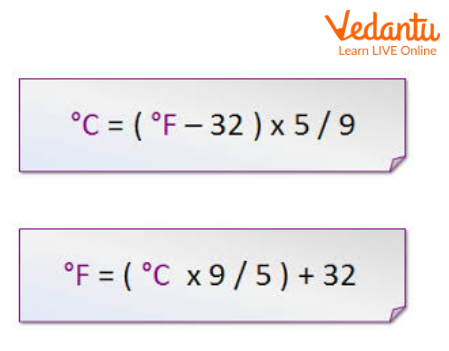Courses
Courses for Kids
Free study material
Free LIVE classes
More

# Fahrenheit to Celsius Formula in CLIVE
Join Vedantu’s FREE Mastercalss

## Introduction

Have you ever wondered why sometimes it is so hot that we prefer cooler drinks and wear shorts and t-shirts while sometimes it is so cold that we are wrapped in clothes from head to toe and are always drinking hot coffee or tea? Why does that happen? Well, the main reason is the change of seasons and because of that the temperature changes.Cold and Hot

Temperature also changes as per the day’s duration. Like it is cooler at night and warmer during the day. We feel comfortable when the temperature is in our comfort range. The normal room temperature is 25 degrees Celsius, so when the temperature is around that range, we feel quite comfortable and outside that range always disturbs our inner peace.

## Units of Temperature

There are five units of temperature overall.

1. Celsius

2. Fahrenheit

3. Kelvin

4. Rankine

5. Reaumur

But the two most common units are Celcius and Fahrenheit. Next, we will learn how to convert Fahrenheit to Celsius.

## How to Convert Fahrenheit to Celsius?

As we all know that one unit can be converted into other units by using a certain formula. Similarly, in order to convert a temperature given in Fahrenheit to a temperature in celsius, we use a certain formula.

Equation to Convert Fahrenheit to Celsius:

$F=\{C \times(\dfrac{9}{5})\}+32$

where

•  C = temperature in degrees Celsius

•  F = temperature in degrees Fahrenheit

We can also convert Celsius to Fahrenheit using the below formula:

$C=(F-32) \times \dfrac{5}{9}$

where

•  C = temperature in degrees Celsius

•  F = temperature in degrees Fahrenheit

The above is the Fahrenheit to Celsius formula.Convert Fahrenheit to Celsius Formula

## Fahrenheit to Celsius Examples

Following are the Fahrenheit to Celsius examples.

Example 1. Convert 100 degrees Fahrenheit to Celsius.

Ans: Using the Fahrenheit to celsius formula, we get

$C=(F-32) \times \dfrac{5}{9}$

$C=(100-32) \times \dfrac{5}{9}$

$C=68 \times \dfrac{5}{9}$

$C=37.77 \text { degree celsius }$

Example 2.  Convert 48 degrees celsius to Fahrenheit.

Ans: We know the formula to convert Celcius to Fahrenheit is

$F=\{C \times(\dfrac{9}{5})\}+32$

$F=48 \times \dfrac{9}{5}+32$

$F=86.4+32$

$F=118.4 \text { degree Fahrenheit }$

Example 3. Convert 104 degrees Fahrenheit to Celsius.

Ans: Using the Fahrenheit to celsius equation, we get

$C=(F-32) \times \dfrac{5}{9}$

$C=(104-32) \times \dfrac{5}{9}$

$C=72 \times \dfrac{5}{9}$

$C=40 \text { degree celsius. }$

## Practice on Questions

1. What is 90 degrees Fahrenheit in Celsius?

Ans: 32.22 degrees Celsius

2. What is 25 degrees Celsius in Fahrenheit?

Ans: 77 degrees Fahrenheit

3. The temperature of a place is 50 degrees Celsius. What will be the temperature in Fahrenheit?

Ans: 122 degrees Fahrenheit

4. The temperature of a person suffering from high fever is 106 degrees Fahrenheit. What will be his temperature in celsius?

Ans:  41.11 degrees Celsius

## Summary

The temperature is extremely important for our day-to-day tasks to go on smoothly. If the temperature is extremely hot, then the daily task becomes difficult to carry out due to hot weather conditions. Similarly, if the temperature is too cold, day-to-day tasks become difficult too. The units of temperature also play major roles. The Standard International (SI) unit of temperature is Celsius. Similarly, other units like Fahrenheit, Kelvin, etc. are equally important.

Last updated date: 15th Sep 2023
Total views: 122.7k
Views today: 1.22k

## FAQs on Fahrenheit to Celsius Formula in C

1. What are F and C in the Fahrenheit to celsius formula and what is the boiling point of water in Celsius and Fahrenheit?

Fahrenheit to celsius is the method to convert a temperature given in Fahrenheit to a temperature in Celsius. The formula to convert a temperature in Fahrenheit to Celsius is

$C=(F-32) \times \dfrac{5 }{9}$

The F in the above formula is the temperature in Fahrenheit and the C in the above formula is the temperature in Celsius.

A boiling point is termed a point at which liquid starts boiling and turns into vapour. It is also the point at which water pressure becomes equal to vapour pressure which allows liquid to get converted into vapour. The boiling point of water is 100 degrees Celsius.

2. When are both Fahrenheit and celsius equal?

As everything in nature remains in balance, similarly the same case applies to temperature units as well. Although there are several units of temperature available in nature, there must be a balance between these units as per nature’s rules. This implies that at some point or the other, Kelvin must be equal to Celsius and Celsius must be equal to Fahrenheit and so on. Since we are here dealing with Fahrenheit and celsius, we will find a temperature at which Celsius and Fahrenheit are equal. To find the temperature when both Fahrenheit and celsius are equal, we use the algebraic form as

$C=F$

$(F-32 \times \dfrac{5}{9})=C \times \dfrac{9}{5}+32$

So on solving the above, we get the answer as -40 degrees celsius.